## Pell Equation

A special case of the quadratic Diophantine Equation having the form(1)

where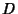is a nonsquare Natural Number. Dörrie (1965) defines the equation as(2)

and calls it the Fermat Difference Equation. The general Pell equation was solved by the Indian mathematician Bhaskara.

Pell equations, as well as the analogous equation with a minus sign on the right, can be solved by finding the Continued Fractionfor. (The trivial solution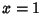,is ignored in all subsequent discussion.) Letdenote theth Convergent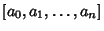, then we are looking for a convergent which obeys the identity(3)

which turns out to always be possible since the Continued Fraction of a Quadratic Surd always becomes periodic at some term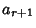, where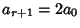, i.e.,(4)

Ifis Odd, thenis Positive and the solution in terms of smallest Integers is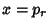and, whereis theth Convergent. Ifis Even, thenis Negative, but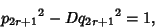(5)

so the solution in smallest Integers is,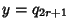. Summarizing,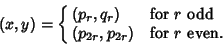(6)

Given one solution(which can be found as above), a whole family of solutions can be found by taking each side to theth Power,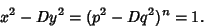(7)

Factoring gives(8)

and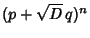(9)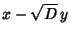(10)

which gives the family of solutions(11)(12)

These solutions also hold for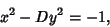(13)

except thatcan take on only Odd values.

The following table gives the smallest integer solutionsto the Pell equation with constant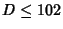(Beiler 1966, p. 254). Square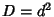are not included, since they would result in an equation of the form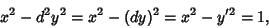(14)

which has no solutions (since the difference of two Squares cannot be 1).2 3 2 54 485 66 3 2 1 55 89 12 5 9 4 56 15 2 6 5 2 57 151 20 7 8 3 58 19603 2574 8 3 1 59 530 69 10 19 6 60 31 4 11 10 3 61 1766319049 226153980 12 7 2 62 63 8 13 649 180 63 8 1 14 15 4 65 129 16 15 4 1 66 65 8 17 33 8 67 48842 5967 18 17 4 68 33 4 19 170 39 69 7775 936 20 9 2 70 251 30 21 55 12 71 3480 413 22 197 42 72 17 2 23 24 5 73 2281249 267000 24 5 1 74 3699 430 26 51 10 75 26 3 27 26 5 76 57799 6630 28 127 24 77 351 40 29 9801 1820 78 53 6 30 11 2 79 80 9 31 1520 273 80 9 1 32 17 3 82 163 18 33 23 4 83 82 9 34 35 6 84 55 6 35 6 1 85 285769 30996 37 73 12 86 10405 1122 38 37 6 87 28 3 39 25 4 88 197 21 40 19 3 89 500001 53000 41 2049 320 90 19 2 42 13 2 91 1574 165 43 3482 531 92 1151 120 44 199 30 93 12151 1260 45 161 24 94 2143295 221064 46 24335 3588 95 39 4 47 48 7 96 49 5 48 7 1 97 62809633 6377352 50 99 14 98 99 10 51 50 7 99 10 1 52 649 90 101 201 20 53 66249 9100 102 101 10

The first few minimal values ofandfor nonsquareare 3, 2, 9, 5, 8, 3, 19, 10, 7, 649, ... (Sloane's A033313) and 2, 1, 4, 2, 3, 1, 6, 3, 2, 180, ... (Sloane's A033317), respectively. The values ofhaving, 3, ... are 3, 2, 15, 6, 35, 12, 7, 5, 11, 30, ... (Sloane's A033314) and the values ofhaving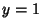, 2, ... are 3, 2, 7, 5, 23, 10, 47, 17, 79, 26, ... (Sloane's A033318). Values of the incrementally largest minimalare 3, 9, 19, 649, 9801, 24335, 66249, ... (Sloane's A033315) which occur at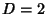, 5, 10, 13, 29, 46, 53, 61, 109, 181, ... (Sloane's A033316). Values of the incrementally largest minimalare 2, 4, 6, 180, 1820, 3588, 9100, 226153980, ... (Sloane's A033319), which occur at, 5, 10, 13, 29, 46, 53, 61, ... (Sloane's A033320).

References

Beiler, A. H. The Pellian.'' Ch. 22 in Recreations in the Theory of Numbers: The Queen of Mathematics Entertains. New York: Dover, pp. 248-268, 1966.

Degan, C. F. Canon Pellianus. Copenhagen, Denmark, 1817.

Dörrie, H. 100 Great Problems of Elementary Mathematics: Their History and Solutions. New York: Dover, 1965.

Lagarias, J. C. On the Computational Complexity of Determining the Solvability or Unsolvability of the Equation.'' Trans. Amer. Math. Soc. 260, 485-508, 1980.

Sloane, N. J. A. Sequences A033313, A033314, A033315, A033316, A033317, A033318, A033319, and A033320 in An On-Line Version of the Encyclopedia of Integer Sequences.'' http://www.research.att.com/~njas/sequences/eisonline.html.

Smarandache, F. Un metodo de resolucion de la ecuacion diofantica.'' Gaz. Math. 1, 151-157, 1988.

Smarandache, F.  Method to Solve the Diophantine Equation.'' In Collected Papers, Vol. 1. Lupton, AZ: Erhus University Press, 1996.

Stillwell, J. C. Mathematics and Its History. New York: Springer-Verlag, 1989.

Whitford, E. E. Pell Equation. New York: Columbia University Press, 1912.